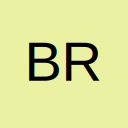# Length of number in a variable

• Hello All

I am writing code that looks at entered employee numbers, but what i need to do is make sure there are 5 numbers in the variable (Number) i.e 12160. i tried using the

Code
``If Len(Number) <> 5 Then``

But all i get is 2 because number is defined as an integer.

any idea's

thank you
Brian G.

[SIZE="6"][FONT="Comic Sans MS"]Brain G.[/FONT][/SIZE] :loopain:

• Re: Length of number in a variable

Brian

Where is the variable Number getting declared and where is it getting a value?

Have you declared ut as an integer?

Boo!:yikes:

• Re: Length of number in a variable

Try casting the number to a string first.
[vba] MsgBox Len(CStr(Number))[/vba]

[h4]Cheers
Andy
[/h4]

• Re: Length of number in a variable

[vba]
If Len(CStr(Number)) <> 5 Then
[/vba]

HTH

Bob

• Re: Length of number in a variable

or

[vba]

If Number < 10000 Or Number > 99999 Then
[/vba]

HTH

Bob

• Re: Length of number in a variable

Once again Andy Pope is the Man!

Thank You

[SIZE="6"][FONT="Comic Sans MS"]Brain G.[/FONT][/SIZE] :loopain:

## Participate now!

Don’t have an account yet? Register yourself now and be a part of our community!